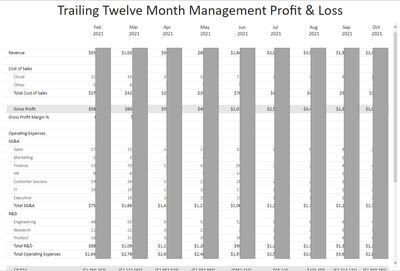cancel
Showing results for
Did you mean:Helper I

## Display data for dates relative to date selected in table

We use Power BI to publish our financial statements (e.g. balance sheet, profit & loss, etc).  Many of our statements also have a trailing 12-month version where it is the current month, and the previous 12 months.  All of the statements are in tables.  I would like to provide a date slicer so the user can select any month, and the financial statements will display for the selected month.  It is correclty presenting the month selected, but I've been unable to determine how to have the trailing months present correctly.  Each of the columns is a measure, which allows me to customize the order of the statement items, insert blank rows, etc.Here is the measure for the selected month (trimmed due to character constraints in this post):

PL3_ActualSwitchMTD =

VAR Revenue = CALCULATE(SUM(Transactions[Net Change]), FILTER(Transactions, Transactions[Year]=[ReportYear]), FILTER(Transactions, Transactions[Month]=[ReportMonth]), FILTER(Transactions, Transactions[Source]="Actual"), FILTER(Mapping, Mapping[PLcalc] = "Revenue"))*-1
VAR COSCloud = CALCULATE(SUM(Transactions[Net Change]), FILTER(Transactions, Transactions[Year]=[ReportYear]), FILTER(Transactions, Transactions[Month]=[ReportMonth]), FILTER(Transactions, Transactions[Source]="Actual"), FILTER(Mapping, Mapping[PLcalc] = "COS - Cloud"))
VAR COSOther = CALCULATE(SUM(Transactions[Net Change]), FILTER(Transactions, Transactions[Year]=[ReportYear]), FILTER(Transactions, Transactions[Month]=[ReportMonth]), FILTER(Transactions, Transactions[Source]="Actual"), FILTER(Mapping, Mapping[PLcalc] = "COS - Other"))
..........

RETURN
SWITCH (
TRUE (),

MAX('ReportConfig'[PLvar3]) = "blank", "",
MAX('ReportConfig'[PLvar3]) = "Revenue", FORMAT(Revenue, "\$#,###;(\$#,###)"),
MAX('ReportConfig'[PLvar3]) = "COSCloud", FORMAT(COSCloud, "#,###;(#,###)"),
MAX('ReportConfig'[PLvar3]) = "COSOther", FORMAT(COSOther, "#,###;(#,###)"),
........
)

ReportMonth = MONTH(SELECTEDVALUE('Date'[MonthEndDate]))
ReportYear = YEAR(SELECTEDVALUE('Date'[MonthEndDate]))

Here is what I tried for the month previous to the selected month, which is erroneously showing the same values as the selected month (trimmed due to character constraints in this post):

TTM MgmtDetailPL-SelectedMonth-1 =

VAR MonthEndDate = SELECTEDVALUE('Date'[MonthEndDate])
VAR RevenueCalc = CALCULATE(SUM(Transactions[Net Change]), FILTER(Transactions, Transactions[Source]="Actual"), FILTER(Mapping, Mapping[PLcalc] = "Revenue"))*-1
VAR Revenue = CALCULATE(RevenueCalc, REMOVEFILTERS('Date'), KEEPFILTERS(SelPeriod), USERELATIONSHIP('Date'[Date], 'Calendar'[Date]))
VAR COSCloudCalc = CALCULATE(SUM(Transactions[Net Change]), FILTER(Transactions, Transactions[Source]="Actual"), FILTER(Mapping, Mapping[PLcalc] = "COS - Cloud"))
VAR COSCloud = CALCULATE(COSCloudCalc, REMOVEFILTERS('Date'), KEEPFILTERS(SelPeriod), USERELATIONSHIP('Date'[Date], 'Calendar'[Date]))
VAR COSOtherCalc = CALCULATE(SUM(Transactions[Net Change]), FILTER(Transactions, Transactions[Source]="Actual"), FILTER(Mapping, Mapping[PLcalc] = "COS - Other"))
VAR COSOther = CALCULATE(COSOtherCalc, REMOVEFILTERS('Date'), KEEPFILTERS(SelPeriod), USERELATIONSHIP('Date'[Date], 'Calendar'[Date]))
........

RETURN
SWITCH (
TRUE (),

MAX('ReportConfig'[PLvar3]) = "blank", "",
MAX('ReportConfig'[PLvar3]) = "Revenue", FORMAT(Revenue, "\$#,###;(\$#,###)"),
MAX('ReportConfig'[PLvar3]) = "COSCloud", FORMAT(COSCloud, "#,###;(#,###)"),
MAX('ReportConfig'[PLvar3]) = "COSOther", FORMAT(COSOther, "#,###;(#,###)"),
........
)

I can't share the .pbix due to the confidential nature of the content.  Does anyone have suggestions how to fix this?

Thanks!
5 REPLIES 5Super User

@StaceyLGriffeth this is pretty difficult to try to debug without any sample data to work with, but the thing that immediately jumps out to me in your second measure are the RevenueCalc/Revenue and COSCloudCalc/COSCloud variables.

I don't think you can reference a variable within a CALCULATE function as you are doing there.  My understanding is that once the "Calc" variables have been declared and evaluated, they are treated as constants in subsequent variables, until a RETURN statement is reached.  That is, they would not be affected by CALCULATE modifiers.

I would recommend combining the "calc" variables into a single step which contains the necessary date filter modifiers to select the previous month.

Enterprise DNA has an excellent video explaining the concept of "variables as constants", which I think is what you are running into here.
What does "Variables Are Constants" Really Mean?Helper I

@ebeery , thank you for taking the time to look over this.  I can confirm that those values do calculate correctly, it is only that they are not calculating for the correct timeframe.Super User

@StaceyLGriffeth Did you watch the video I linked? I think it describes exactly what you are seeing here... Let me try to explain a different way...

Take your Revenue variable for example:

``VAR Revenue = CALCULATE(RevenueCalc, REMOVEFILTERS('Date'), KEEPFILTERS(SelPeriod), USERELATIONSHIP('Date'[Date], 'Calendar'[Date]))``

The "KEEPFILTERS" modifier is what is supposed to be adjusting the timeframe.  But it has no effect, because "RevenueCalc" is treated as a constant, and therefore cannot be affected by a CALCULATE modifier.  You need to combine the Revenue and RevenueCalc variables into a single variable (and same with your COSCloud and COSOther variables).Helper I

@ebeery Thank you so much for the clarification, I understood that better than I did the video.  I've adjusted the calculations to be:

VAR MonthEndDate = SELECTEDVALUE('Date'[MonthEndDate])
VAR Revenue = CALCULATE(SUM(Transactions[Net Change]), FILTER(Transactions, Transactions[Source]="Actual"), FILTER(Mapping, Mapping[PLcalc] = "Revenue")*-1, REMOVEFILTERS('Date'), KEEPFILTERS(SelPeriod), USERELATIONSHIP('Date'[Date], 'Calendar'[Date]))
VAR COSCloud = CALCULATE(SUM(Transactions[Net Change]), FILTER(Transactions, Transactions[Source]="Actual"), FILTER(Mapping, Mapping[PLcalc] = "COS - Cloud"), REMOVEFILTERS('Date'), KEEPFILTERS(SelPeriod), USERELATIONSHIP('Date'[Date], 'Calendar'[Date]))
VAR COSOther = CALCULATE(SUM(Transactions[Net Change]), FILTER(Transactions, Transactions[Source]="Actual"), FILTER(Mapping, Mapping[PLcalc] = "COS - Other"), REMOVEFILTERS('Date'), KEEPFILTERS(SelPeriod), USERELATIONSHIP('Date'[Date], 'Calendar'[Date]))
.....

RETURN
SWITCH (
TRUE (),

MAX('ReportConfig'[PLvar3]) = "blank", "",
MAX('ReportConfig'[PLvar3]) = "Revenue", FORMAT(Revenue, "\$#,###;(\$#,###)"),
MAX('ReportConfig'[PLvar3]) = "COSCloud", FORMAT(COSCloud, "#,###;(#,###)"),
MAX('ReportConfig'[PLvar3]) = "COSOther", FORMAT(COSOther, "#,###;(#,###)"),
......
)

Now I'm getting the error:

A function 'FILTER' has been used in a True/False expression that is used as a table filter expression. This is not allowed.

Do you think this error is to do with the SelPeriod variable?  I used this approach (remove date filters, keep selperiod filter) in another BI report and it worked correctly.  The only difference between the two is the addition of the AdjMonth variable in this report.  Do I need to combine the AdjMonth and SelPeriod variables into one?

Thanks so much for your help!Super User

@StaceyLGriffeth again this is very difficult to debug without sample data or properly formatted code, but the Revenue variable jumps out to me as not looking quite right.  You're multiplying a Filter argument by -1, when I think you're meaning to multiply the result of the CALCULATE statement by -1.

``VAR Revenue = CALCULATE(SUM(Transactions[Net Change]), FILTER(Transactions, Transactions[Source]="Actual"), FILTER(Mapping, Mapping[PLcalc] = "Revenue")*-1, REMOVEFILTERS('Date'), KEEPFILTERS(SelPeriod), USERELATIONSHIP('Date'[Date], 'Calendar'[Date]))``

Putting this in the proper format using https://www.daxformatter.com/ makes this more clear:

``````VAR Revenue =
CALCULATE (
SUM ( Transactions[Net Change] ),
FILTER ( Transactions, Transactions[Source] = "Actual" ),
FILTER ( Mapping, Mapping[PLcalc] = "Revenue" ) * -1,
REMOVEFILTERS ( 'Date' ),
KEEPFILTERS ( SelPeriod ),
USERELATIONSHIP ( 'Date'[Date], 'Calendar'[Date] )
)``````